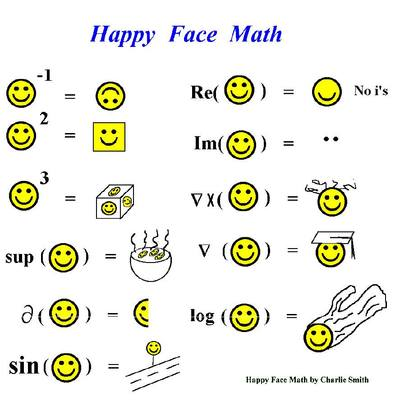Factoring Polynomials
4 years ago
mrrileysmath
Save
Edit
Host a game
Live GameLive
Homework
Solo Practice
Practice10 QuestionsShow answers
• Question 1
120 secondsQ. Factor completely:
16x2 - 20xy
4 (4x2 - 5xy)
4x (4x - 5y)
4x2 (4 - 5y)
2(duck)
• Question 2
180 secondsQ. Factor completely:
4x2y - 12xy + 5y
(2xy - 1) (2x - 5)
prime
y (2x - 1) (2x - 5)
4 (xy - 5) (x - 1)
• Question 3
180 secondsQ. Factor completely:
y2 - 7y + 12
(y - 3) (y - 4)
(y + 3) (y - 4)
(y + 3) (y + 4)
(y - 2) (y - 6)
• Question 4
180 secondsQ. Factor completely:
2x2 + 7x + 6
(2x - 3) (x - 2)
(2x + 3) (x + 2)
(x + 3) (2x + 2)
prime
• Question 5
180 secondsQ. Factor completely:
4x2 - 121
(2x + 11) (11 - 2x)
(2x - 11) (2x - 11)
(2x + 11) (2x + 11)
(2x + 11) (2x - 11)
• Question 6
180 secondsQ. Factor completely:
x2 - 5x + 3
(x + 3) (x + 1)
(x - 3) (x - 1)
prime
(x - 3) (x + 1)
• Question 7
180 secondsQ. Factor completely:
-8x2 + 10x + 3
(2x - 3) (-4x - 1)
-1 (2x - 3) (4x + 1)
-1 (-2x + 3) (4x + 1)
(-2x + 3) (4x + 1)
• Question 8
180 secondsQ. Factor completely:
169 - 9x2
-1(3x + 13) (3x - 13)
(3x + 13) (3x - 13)
(3x + 13) (-3x - 13)
-1(3x - 13) (3x - 13)
• Question 9
300 secondsQ. The area of a circular rug is 6πk2 + 16πk + 8π
Factor the expression completely.
π (6k +4)(k + 2)
2π (3k - 2)(k - 2)
2π (k + 2)(3k + 2)
π (2k +4)(3k + 2)
• Question 10
300 secondsQ. Factor completely:
49p2 + 84p + 36